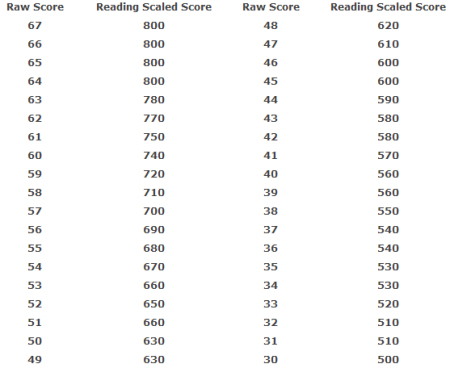# 2015年SAT数学应试经典练习试题

2014-12-12 10:44:00 来源：无忧考网
1.试题

1. If 10+x is 5 more than 10, what is the value of 2x ?

(A)-5 (B) 5 (C) 10 (D) 25 (E) 50

2. If(x -2)2 =49, then x could be

(A) -9 (B) -7 (C) 2 (D) 5 (E) 9

3. If this page was folded along the dotted line in the figure above, the left half of the letter W would exactly coincide with the right half of W. Which of the following letters, as shown, CANNOT be folded along a vertical line so that its left half would coincide with its right half?

(A) A (B) I (C) O (D) U (E) E

4. In a certain store, the regular price of a refrigerator is \$600. How much money is saved by buying this refrigerator at 20 percent off the regular price rather than buying it on sale at 10 percent off the regular price

with an additional discount of 10 percent off the sale price?

(A) \$6 (B) \$12 (C) \$24 (D) \$54 (E) \$60

5. Which of the following is the graph of a function f such that f(x) = 0 for exactly two values of x between

-5 and 5 ?

(A) (B)

(C) (D)

(E)

6. Based on the portions of the graphs of the functions f and g shown above, what are all values of x between -6 and 6 for which g x f x ?

(A) -6 < x <-3 only (B) -3 < x < 0 only (C) 0 < x < 3 only

(D) 3 < x < 6 only (E) -6 < x < -3 and 0 < x < 3。

7. Point O is the center of both circles in the figure above. If the circumference of the large circle is 36 and the radius of the small circle is half of the radius of the large circle, what is the length of the darkened arc?

(A) 10 (B) 8 (C) 6 (D) 4 (E) 2

8. If x = 20 and y = 30 in the figure above, what is the value of z ?

(A) 60 (B) 70 (C) 80 (D) 90 (E) 100

9. If 6 < |x-3| < 7 and x < 0, what is one possible value of |x| ?

10. What is the product of the smallest prime number that is greater than 50 and the greatest prime number that is less than 50 ?

2.答案

1.(C)， 2. (E)， 3. (E)， 4.(A)， 5.(D)， 6. (B)， 7. (D)， 8. (B)， 9.x < 4, 10.1300

第二节SAT阅读

SAT的阅读部分对中国学生来说是最难对付的了，一是它的词汇量要求极大；二是它对时间的要求甚严，三是它的问题中有一些即使翻译成汉语也是很不好回答的。

SAT阅读部分由3个试卷共65道选择题组成，均是选择试题。每道选择题有五项选择答案，总测试时间为70分钟。下表是阅读试题的一个得分换算表，尽管每次考试的得分表略有不同，但不会有大的变化。表中左面的一列是原始分数(R=C-W)，右面的一列为最终得分。

表5-2-1 SAT阅读考试的原始分数和总分数的换算表这里，C是英文Correct的缩写，它代表答对的题目数；W是英文Wrong的缩写，代表答错的多项选择题数乘以1/4后再把结果四舍五入后得到的值。
如果一位考生答对了65道题，错了2道题，那么他的C =65，W=1(2*1/4=0.5, 四舍五入)，所以的R=C-W=65-1=64；由上表得知该考生的SAT阅读部分的得分是满分(800分)。由此可见在SAT考试中尽管你错了题，但仍然可能得满分。

SAT试题相关推荐京公网安备 11010802026788号